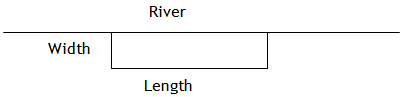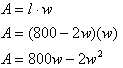Area Problems

Because area is modeled by square units, all area problems can be modeled by quadratic functions.

Example A rancher needs to construct a rectangular stock pen, using the local creek as one of the sides. The other three sides can be constructed out of 800 meters of fencing material (see the diagram below). He can make the stock pen a variety of shapes - long and thin, short and fat, square or any shape in between. Create a quadratic equation and graph that can be used to determine which rectangular shape will give him the maximum area. Then answer the following questions: What is the domain and range? For what values of length is the area zero? For what value of length is there a maximum area?Step 1 . Determine an expression for the length and the width of the pen.

The perimeter of the rectangular pen has the formula, P = 2w + 1l. Since there is 800 feet of fence to use, the formula for the perimeter is 800 = 2w + l. Solve this formula for l to find an expression that will represent the length of the fence.

2w + l = 800

l = 800 - 2w

Step 2. Determine a quadratic equation that will model the area of the pen.